Modal logic

A modal logic was originally designed to describe the logical relations of modal notions. The list of the notions includes metaphysical modalities (necessities, possibilities, etc.), epistemic modalities (knowledge, belief, etc.), temporal modalities (future, past, etc.), and deontic modalities (obligation, permission, etc.). Because of the importance of these modal notions, modal logics have attracted many areas in philosophy, including metaphysics and epistemology. However, the interests in modal logics are not limited to such philosophical investigations. Because of its wide applicability, the general framework of modal logic have been used in various areas such as artificial intelligence, database theory, and game theory.

The languages of modal logics usually extend preexisting logics, e.g propositional logic, first-order logic with modal operators, which are often symbolized as boxes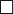$\Box$ and diamonds$\Diamond$. Semantic structures for the languages of modal logics are relational structures and the modal languages can be characterized as describing various properties of the relational structures.

Basic Ideas

One major notion that has been considered in modal logics is metaphysical modality. Examples of the modal notion are necessity and possibility. The modal logic that describe the logical relations of statements such as “It is necessary that 2+2=4,” “It is possible that Bigfoot exists” etc. is called alethic modal logic. The main idea of analyzing such modal statements was produced based on the metaphysical view that is usually credited to Leibniz. The idea is to analyze the statement of the form “It is necessary that p” as “In all possible worlds, p is the case,” and “It is possible that p” as “There is some possible world in which p is the case.” In other words, necessity is analyzed as the truth in all possible worlds, and possibility, as the truth in some possible world.

Based on this idea, alethic modal logic clarifies the logical relations of modal statements of the kind in question. For instance, one basic equivalence in alethic modal logic, the one between “It is necessary that p” and “It is not possible that not-p,” is explicated as the equivalence between “In all possible worlds, p is the case” and “There is no possible world in which p is not the case.” Alethic modal logic enables one to see more complex relations of the metaphysical modal statements.

This general idea is modeled in what is called Kripke semantics by relational structures (see below). Because of the wide applicability of the general framework, modal logics have been used, beyond the formalization of metaphysical modality, to represent modal concepts and phenomena. Depending on the purposes of applications, modal logics get specific names. Epistemic logic is designed to describe epistemic notions such as knowledge and belief; temporal logic, temporal structures; deontic logic, deontic notions such as obligation and permission; dynamic logic, actions of computer programs, etc.

Standard Syntax and Semantics of Modal Logics

Syntax

The languages of modal logics extend preexisting logical languages with modal operators—most standard boxes$\Box$ and diamonds$\Diamond$. The intended meanings of boxes and diamonds, say, in alethic modal logic, are respectively “It is necessary that...” and “It is possible that....”

The language of propositional modal logic—the extension of propositional logic with modal operators—consists of propositional variables (p, q, r, …), Boolean connectives ($\lnot$,$\wedge$,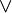$\vee$,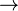$\rightarrow$), and modal operators ($\Box$ and$\Diamond$). In a standard way, the sentences of propositional modal logic is recursively defined as follows: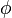$\phi$ := p (with p a propositional variable) |$\phi \wedge \psi$ |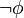$\lnot \phi$ |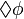$\Diamond \phi$

The other Boolean connectives are defined as usual (for instance, "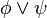$\phi \vee \psi$" is defined as "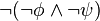$\lnot (\lnot \phi \wedge \lnot \psi)$" and "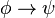$\phi \rightarrow \psi$," as "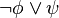$\lnot \phi \vee \psi$"), and, based on the observation about the above basic equivalence, “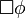$\Box\phi$” is defined as the abbreviation of “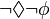$\lnot\Diamond\lnot\phi$.”

Other than the language of modal propositional logic, there are various versions of extensions of preexisting languages. Extensions with modal operators are considered for other preexisting languages. For instance, the extension of first-order logic, called modal predicate logic, has been widely considered. Also, extensions are given with modality operators with multiple arities, i.e. modal operators that are followed by a multiple number of formulas rather than by just a single formula as is the case of the propositional modal logic presented above.

Kripke Semantics

The standard semantics of modal languages is Kripke semantics, which is given by relational models. The Kripke semantics of propositional modal logic can be presented as follows. A frame is a tuple (W, R), where W is an non-empty set and R is a two-place relation on W. W can be thought of as a set of possible world, and R, the accessibility relation between worlds, which represents the possible worlds that are considered at a given world, i.e. if we are at a world$w_0$, every possible world v such that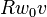$Rw_0v$ represents the possibility that are considered at a world$w_0$. Given a frame (W, R), a model is a tuple (W, R, V) where V is a map that assigns to a world a valuation function on propositional variables, i.e. for a given world w, V(w) is a function from the set of propositional variables to {0, 1} where 0 and 1 represents the truth-values, False and True. Truth of formulas is defined with respect to a model M and a world w as follows:

(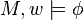$M, w\models \phi$ reads as “$\phi$ is true at a world$\phi$ in a model M.)

•$M, w \models p$ iff V(w)(p)=1 (with p a propositional variable)
•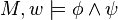$M, w \models \phi \wedge \psi$ iff$M,w\models \phi$ and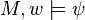$M, w\models \psi$.
•$M, w \models \lnot \phi$ iff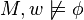$M, w\not\models \phi$.
•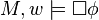$M, w\models \Box \phi$ iff, for every world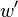$w^\prime$ such that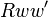$Rww^\prime$,$M, w\models \phi$.

The last clause captures the main idea of Leibnizian conception of necessary truth as truth in all possibilities in such a way that “It is necessary that$\phi$” is true at a world w in a model M if and only if$\phi$ is true in all possible worlds accessible from a world w.

A sentence is valid in a model M if it is true at every possible world in M. A sentence is valid in a frame F if it is valid in every model based on F. A sentence is valid if it is valid in all frames (or every model).

By extending this model-theoretic framework, the semantics for other modal languages are given. In modal predicate logic, a model is designed so that a domain of quantification is associated with each possible world, and in modal logics with modal operator with multiple arities, the accessibility relations of appropriate arities on possible worlds are taken.

Axiomatic Systems and Frame Correspondence

The Kripke semantics presented here has a sound and complete axiomatic system, i.e. the system in which, for a sentence$\phi$,$\phi$ is valid if and only if$\phi$ is provable. The system is called K. K is the system obtained by adding the following two principles to propositional logic:

Necessitation Rule: If A is a theorem,$\Box A$ is a theorem.

K:$\Box (\phi \rightarrow \psi) \rightarrow (\Box \phi \rightarrow \Box \psi)$

Various systems are obtained by adding extra axioms to K. Some of the most famous axioms are:

T: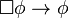$\Box \phi \rightarrow \phi$

S4: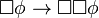$\Box \phi \rightarrow \Box \Box \phi$

S5:$\Diamond \phi \rightarrow \Box \Diamond \phi$

The system T is obtained by adding the axiom scheme T to K. T is sound and complete with respect to the set of models that are based on reflexive frames (i.e. frames (W, R) such that, for all x in W, Rxx). The addition of S4 to T yields the system S4. S4 is sound and complete with respect to reflexive and transitive frames (Transitive frames are frames (W, R) such that, for all x, y, z in W, if Rxy and Ryz, then Rxz). Finally, the addition of S5 to S4 yields the system S5, which is sound and complete with respect to reflexive, transitive and symmetric frames, (symmetric frames are frames (W, R) such that, for every x, y in W, if Rxy, then Ryx.

Some Applications

Modal logics have been applied to capture various kinds of concepts and phenomena. Depending on the subject matter, modal operators are interpreted in different ways. Here are some of the major examples:

Epistemic Logic: Boxes are written as “K” or “B.” “K$\phi$” is interpreted as “It is known that$\phi$,” and “B$\phi$,” as “It is believed that$\phi$.”

Deontic Logic: Boxes and diamonds are written as “O” and “P” respectively. “O$\phi$” is interpreted as “It is obligatory that$\phi$,” and “P$\phi$,” as “It is permitted that$\phi$.”

Temporal Logic: Boxes are written as “G” for the future and “H” for the past. “G$\phi$” means "$\phi$ will be always the case” and “H$\phi$,” “$\phi$ was always the case.” The corresponding diamonds are written as “F” and “P” respectively. “F$\phi$” and “P$\phi$” mean “It will be the case that$\phi$” and “It was the case that$\phi$.”

Depending on the interpretations of modalities, different axiomatic constraints are placed on modal operators. For instance, in epistemic logic, it is appropriate to accept the T axiom, since the knowledge that$\phi$ implies that$\phi$ is true; however, in deontic logic, T might not be appropriate, since$\phi$ might not be the case even if it is obligatory that$\phi$. There have been wide ranges of discussions about what axioms are appropriate for each modal notions.

A Brief Historical Note on Modal Logic

Although Aristotle's logic is almost entirely concerned with the theory of the categorical syllogism, his work also contains some extended arguments on points of modal logic (such as his famous Sea-Battle Argument in De Interpretatione § 9) and their connection with potentialities and with time. Following on his works, the Scholastics developed the groundwork for a rigorous theory of modal logic, mostly within the context of commentary on the logic of statements about essence and accident. Among the medieval writers, some of the most important works on modal logic can be found in the works of William of Ockham and John Duns Scotus.

The start of formal modal logics is usually associated with the work by C. I. Lewis, who introduced a system (later called S3) in his monograph A Survey of Symbolic Logic (1918) and (with C. H. Langford) the systems S1-S5 in the book Symbolic Logic (1932). The first formalization of deontic logic was by E. Mally in 1920s. Epistemic logic was initiated by G. H. von Wright and further developed by J. Hintikka in 50s and 60s. Temporal logic was developed by A. N. Prior in 1960s. The relational semantics for modal logic was developed in the works by J. Hintikka, Kanger, and Kripke in late 50s and early 60s.